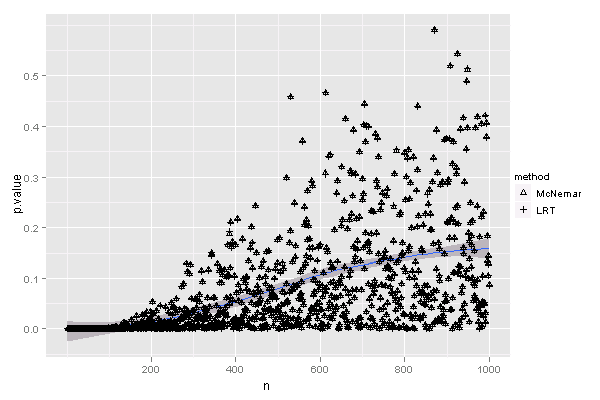# 渐近理想国：McNemar检验的两种统计量

### 谢益辉 2010-05-19

``````set.seed(123)
nmax = 1000
p = matrix(nrow = nmax, ncol = 2)
for (n in 2:nmax) {
# 生成服从二项分布的随机数，分别计算两种统计量并作KS检验、记录P值
b = rbinom(500, n, 0.5)
x1 = (b - (n - b))^2/n
x2 = 2 * b * log(2 * b/n) + 2 * (n - b) * log(2 * (n - b)/n)
p[n, 1] = ks.test(x1, "pchisq", df = 1)\$p.value
p[n, 2] = ks.test(x2, "pchisq", df = 1)\$p.value
}
# 调整一下数据格式，画图：随着n增大，P值如何变化？
library(ggplot2)
d = melt(p, varnames = c("n", "method"))
d\$method = factor(d\$method, labels = c("McNemar", "LRT"))
colnames(d) = "p.value"
qplot(n, p.value, data = d, shape = method, geom = c("smooth", "point")) +
scale_shape_manual(values = c(2, 3))
``````